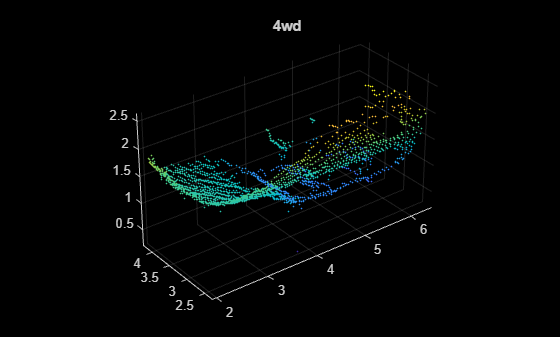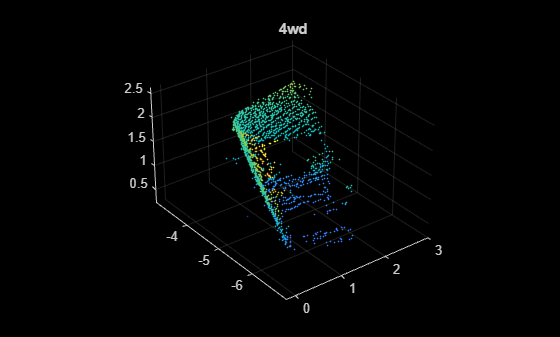Augment Point Cloud Data For Deep Learning

This example demonstrates how to setup a basic randomized data augmentation pipeline when working with point cloud data in deep learning based workflows. Data augmentation is almost always desirable when working with deep learning because it helps to reduce overfitting during training and can add robustness to types of data transformations which may not be well represented in the original training data.

Import point cloud data

dataOut = preview(dsTrain)
dataOut=1×2 cell array
{1×1 pointCloud}    {[4wd]}

The datastore dsTrain yields a pointCloud object and an associated scalar categorical label for each observation.

figure
pcshow(dataOut{1});
title(dataOut{2});Define augmentation pipeline

The transform function of a datastore is a convenient tool for defining augmentation pipelines.

dsAugmented = transform(dsTrain,@augmentPointCloud);

The augmentPointCloud function shown below, applies randomized rotation, homogenous scale, randomized reflection across the x- and y-axes, and randomized per point jitter to each observation using the randomAffine3d function to construct randomized affine transformations and the pctransform function to apply these transformations to each input point cloud.

dataOut = preview(dsAugmented)
dataOut=1×2 cell array
{1×1 pointCloud}    {[4wd]}

It is always a good idea to visually inspect the data that comes out of any augmentation that is done on training data to make sure that the data looks as expected. The point cloud below is the same as the original shown previously, but with randomized affine warping with per point jitter added.

figure
pcshow(dataOut{1});
title(dataOut{2});The resulting TransformedDatastore and dsAugmented can be passed to deep learning functions including trainNetwork, predict, and classify for use in training and inference.

Supporting Functions

if nargin == 0
dataLoc = pwd();
end

dataLoc = string(dataLoc);

url = "http://www.acfr.usyd.edu.au/papers/data/";
name = "sydney-urban-objects-dataset.tar.gz";

if ~exist(fullfile(dataLoc,'sydney-urban-objects-dataset'),'dir')
untar(url+name,dataLoc);
end

datasetPath = dataLoc.append('sydney-urban-objects-dataset');

end

% loadSydneyUrbanObjectsData Datastore with point clouds and
% associated categorical labels for Sydney Urban Objects dataset.
%
% ds = loadSydneyUrbanObjectsData(datapath) constructs a datastore that
% represents point clouds and associated categories for the Sydney Urban
% Objects dataset. The input, datapath, is a string or char array which
% represents the path to the root directory of the Sydney Urban Objects
% Dataset.
%
% ds = loadSydneyUrbanObjectsData(___,folds) optionally allows
% specification of desired folds that you wish to be included in the
% output ds. For example, [1 2 4] specifies that you want the first,
% second, and fourth folds of the Dataset. Default: [1 2 3 4].

if nargin < 2
folds = 1:4;
end

datapath = string(datapath);
path = fullfile(datapath,'objects',filesep);

% For now, include all folds in Datastore
foldNames{1} = importdata(fullfile(datapath,'folds','fold0.txt'));
foldNames{2} = importdata(fullfile(datapath,'folds','fold1.txt'));
foldNames{3} = importdata(fullfile(datapath,'folds','fold2.txt'));
foldNames{4} = importdata(fullfile(datapath,'folds','fold3.txt'));
names = foldNames(folds);
names = vertcat(names{:});

fullFilenames = append(path,names);

end

function dataOut = extractTrainingData(fname)

[~,name] = fileparts(fname);
name = string(name);
name = extractBefore(name,'.');

labelNames = ["4wd","bench","bicycle","biker",...
"building","bus","car","cyclist","excavator","pedestrian","pillar",...
"pole","post","scooter","ticket_machine","traffic_lights","traffic_sign",...
"trailer","trash","tree","truck","trunk","umbrella","ute","van","vegetation"];

label = categorical(name,labelNames);

dataOut = {pointCloud(pointData,'Intensity',intensity),label};

end

% readbin Read point and intensity data from Sydney Urban Object binary
% files.

% names = ['t','intensity','id',...
%          'x','y','z',...
%          'azimuth','range','pid']
%
% formats = ['int64', 'uint8', 'uint8',...
%            'float32', 'float32', 'float32',...
%            'float32', 'float32', 'int32']

fid = fopen(fname, 'r');
c = onCleanup(@() fclose(fid));

fseek(fid,10,-1); % Move to the first X point location 10 bytes from beginning
fseek(fid,14,-1);
fseek(fid,18,-1);

fseek(fid,8,-1);

pointData = [X,Y,Z];

end

function dataOut = augmentPointCloud(data)

ptCloud = data{1};
label = data{2};

% Apply randomized rotation about Z axis.
tform = randomAffine3d('Rotation',@() deal([0 0 1],360*rand),'Scale',[0.98,1.02],'XReflection',true,'YReflection',true); % Randomized rotation about z axis
ptCloud = pctransform(ptCloud,tform);

% Apply jitter to each point in point cloud
amountOfJitter = 0.01;
numPoints = size(ptCloud.Location,1);
D = zeros(size(ptCloud.Location),'like',ptCloud.Location);
D(:,1) = diff(ptCloud.XLimits)*rand(numPoints,1);
D(:,2) = diff(ptCloud.YLimits)*rand(numPoints,1);
D(:,3) = diff(ptCloud.ZLimits)*rand(numPoints,1);
D = amountOfJitter.*D;
ptCloud = pctransform(ptCloud,D);

dataOut = {ptCloud,label};

end